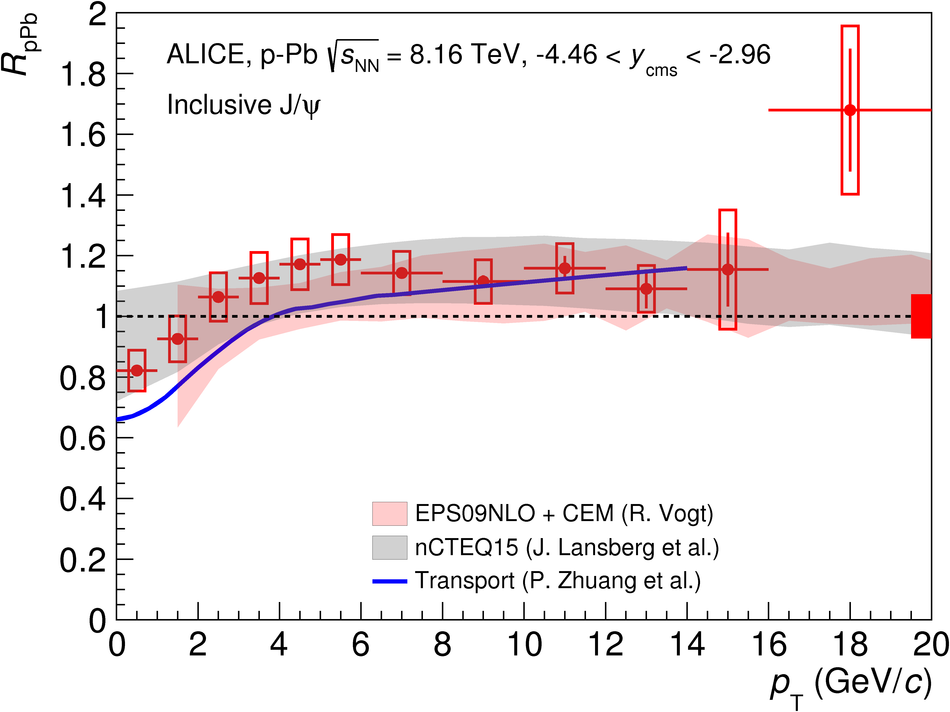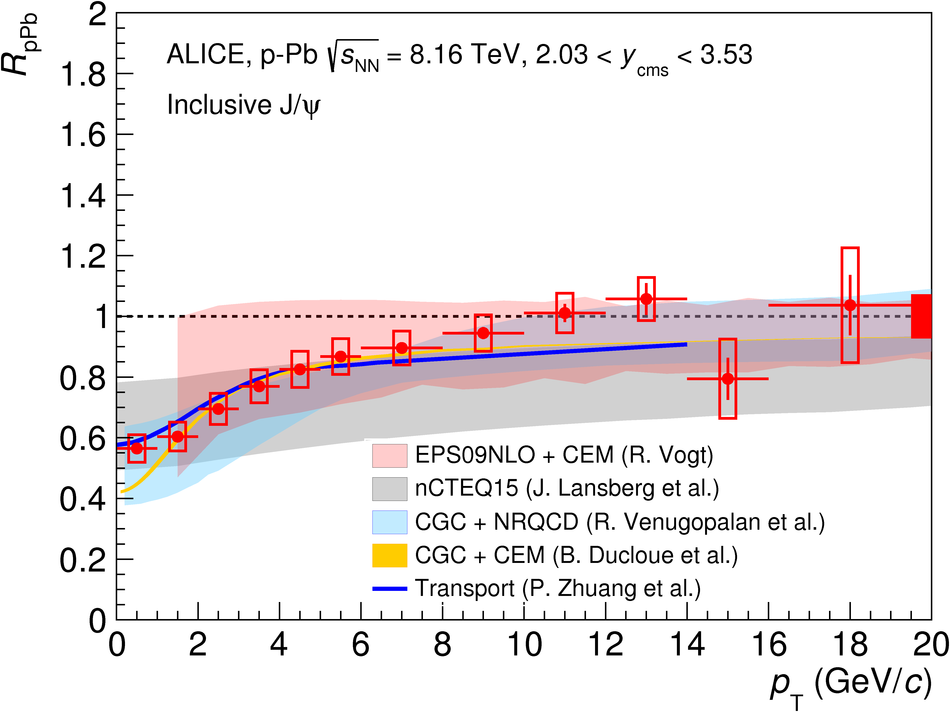Figure 7

 Comparison of the ALICE results on the $p_{\rm T}$-dependence of the inclusive J/$\psi$ nuclear modification factors in \mbox{Pb--p} (left) and \mbox{p--Pb} (right) collisions at $\sqrt{s_{\rm NN}}=8.16$ TeV with model calculations~ (see text for details). The horizontal bars on the experimental points correspond to the bin size. The vertical error bars represent the statistical uncertainties, the boxes around the points the uncorrelated systematic uncertainties and the filled box around unity the correlated uncertainties.